# Chaos game Sierpinski triangle

Martin McBride
2019-07-17

A chaos game is a simple system for generating an approximation to a particular fractal. It relies on a collection of rules that transform one point (x, y) into a different point (x', y').

The game proceeds like this:

1. Pick an initial point (x, y)
2. Choose one of the rules at random to generate (x', y')
3. Replace the current (x, y) with the transformed (x', y')
4. Mark the pixel at (x, y)
5. Repeat from step 2, for a fixed number of iterations. picking P at random each time

## Creating a Sierpinski triangle

Creating a Sierpinski triangle is quite simple using this method.

We first define 3 points, A, B and C that make up the corners of the triangle. For an equilateral triangle with sides of length 2, centred on the origin, the coordinates of the vertices are shown below (0.866 is the sine of 60 degrees):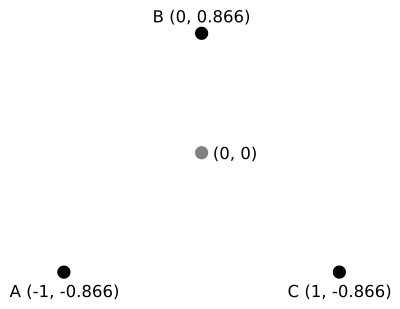The process then works as follows:

1. Pick an initial point (x, y) that is within the triangle - (0, 0) works well
2. Choose one of the points A, B or C at random. (x', y') is the point halfway between the existing (x, y) and the chosen vertex
3. Replace the current (x, y) with the transformed (x', y')
4. Mark the pixel at (x, y)
5. Repeat from step 2, for a fixed number of iterations

Here are the first three iterations:Iteration 1 - let's suppose the first randomly selected vertex is B. We can then draw point 1 halfway between B and the initial point (0, 0).

Iteration 2 - suppose the next randomly selected vertex is C. We can then draw point 2 halfway between C and the previous point 1.

Iteration 3 - suppose the next randomly selected vertex is A. We can then draw point 3 halfway between A and the previous point 2.And so on...

At this point, it is easier to do things in code. After 1000 iterations things are just beginning to take shape:If you continue for 100,000 iterations you get something resembling a Sierpinski triangle: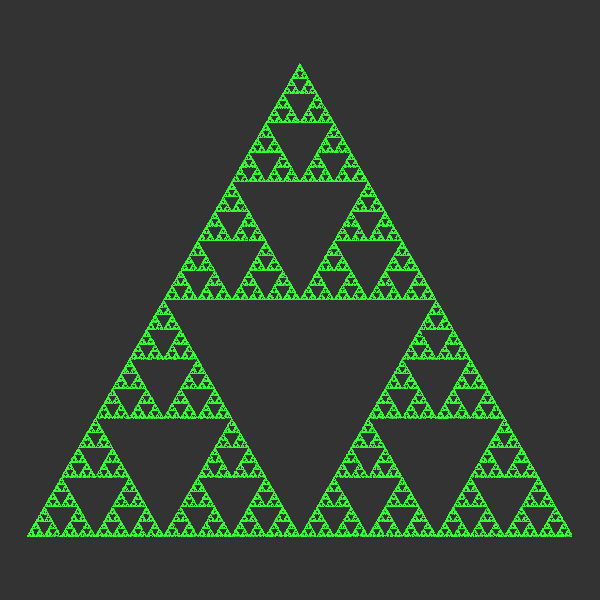Note that this is only an approximation to the triangle. But this technique can be used to create some very interesting patterns.

## The code

Here is the code:

from generativepy.bitmap import makeBitmapImage
from generativepy.color import Color
import math
import random

SIN60 = math.sin(math.pi/3)

SIZE = 600
RANGE = 1.1
ITERATIONS = 100000
VERTICES = [(-1, SIN60),
(0, -SIN60),
(1, SIN60)]

def draw(array, scaling):
x, y = 0, 0
array[:,:] = (0.2, 0.2, 0.2)
color = Color('green').getRGB()
for i in range(ITERATIONS):
vertex = random.choice(VERTICES)
x = (x + vertex)/2
y = (y + vertex)/2
u, v = scaling.user2page((x, y))
array[u, v] += color
return array

makeBitmapImage("chaos-game-sierpinski.png", draw, pixelSize=(SIZE, SIZE),
startX=-RANGE, startY=-RANGE,
width=RANGE*2, height=RANGE*2)


Taking the makeBitmapImage call first, we create a square image 600 pixels square. We set the user coordinates to extend from -1.1 to +1.1 is both directions, so our triangle will fit nicely. User coordinates allow us to work in our chosen dimensions regardless of the actual pixel size of the image, see later.

makeBitmapImage calls our draw function. It passes a 3 dimensional numpy array with shape 600 by 600 by 3 - that is a 600 pixel square image where each pixel has a red, green and blue value. It also passes a BitmapScaling object that lest us translate between pixels and user coordinates.

The first part of the draw function sets things up:

    x, y = 0, 0
array[:,:] = (0.2, 0.2, 0.2)
color = Color('green').getRGB()


Our initial point (x, y) is (0, 0).

The second line uses numpy broadcasting to set the three values of every pixel to dark grey (rgb value (0.2, 0.2, 0.2)). Broadcasting is just an efficient vale to fill a whole array with a single value.

The final line sets color to a tuple containing the rgb values of the colour green.

Next we have the main loop:

    for i in range(ITERATIONS):
vertex = random.choice(VERTICES)
x = (x + vertex)/2
y = (y + vertex)/2
u, v = scaling.user2page((x, y))
array[u, v] += color


We iterate 100,000 times. Each time through we:

• pick a random vertex
• calculate the point halfway betweem the chosen vertex and the previous point (x, y)
• convert the point (x, y), which is in user coordinates into a pixel position (u, v). The function scaling.user2page does this. The result is a pair of integer values that select a particular pixel
• We set the pixel at (u, v) to green by setting the array value

## Chaos game with a square

You might wonder what sort of fractal might we get if we used a square instead of a triangle?

It is easy enough to try, we could simply replace VERTICES with the four corners of a square. Unfortunately the results are disappointing. Instead of a fractal, you get a square full of random noise.

This improve if you add exclusions. For example, we could make an additional rule that when you pick a random vertex, it isn't allowed to be the same one you used last time. So if you used vertex B previously, you must choose between A, C or D next time. Here is the result: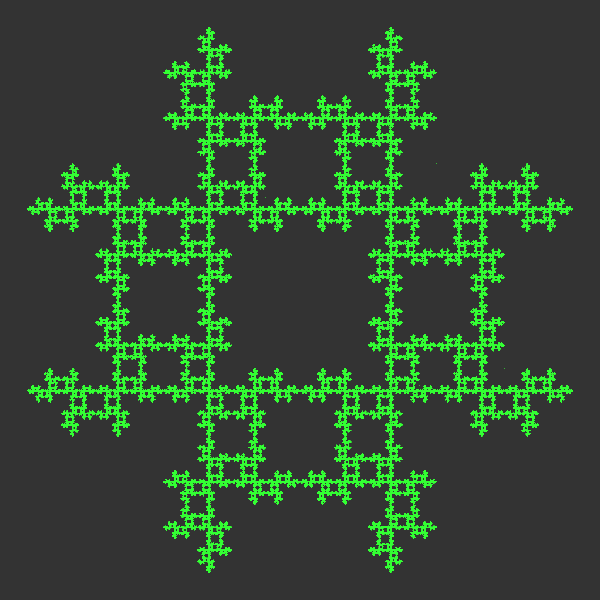And here is the Python code:

from generativepy.bitmap import makeBitmapImage
from generativepy.color import Color
import math
import random

SIZE = 600
RANGE = 1.1
ITERATIONS = 1000000
VERTICES = [(-1, -1),
(-1, 1),
(1, 1),
(1, -1)]
ALLOWED = [0, 1, 1, 1]
COUNT = len(VERTICES)

def draw(array, scaling):
x, y = 0, 0
array[:,:] = (0.2, 0.2, 0.2)
color = Color('green').getRGB()
oldn = 0
for i in range(ITERATIONS):
n = random.randrange(COUNT)
diff = (n - oldn) % COUNT
if ALLOWED[diff]:
vertex = VERTICES[n]
x = (x + vertex)/2
y = (y + vertex)/2
u, v = scaling.user2page((x, y))
array[u, v] += color
oldn = n
return array

makeBitmapImage("chaos-game-square.png", draw, pixelSize=(SIZE, SIZE),
startX=-RANGE, startY=-RANGE,
width=RANGE*2, height=RANGE*2)


Here we have changed the VERTICES to be the four corners of a square. The ALLOWED list indicates which vertices are permitted. The list starts at the previous vertex and cycles round. So for example if the previous vertex was B, the allowqed list [0, 1, 1, 1] would refer to vertices B, C, D, A. So the previous vertex B would be excluded, all others allowed.

Here is the main loop:

    for i in range(ITERATIONS):
n = random.randrange(COUNT)
diff = (n - oldn) % COUNT
if ALLOWED[diff]:
vertex = VERTICES[n]
x = (x + vertex)/2
y = (y + vertex)/2
u, v = scaling.user2page((x, y))
array[u, v] += color
oldn = n


In this case we pick a number n in the range 0 to COUNT-1 (COUNT is the number of vertices). This represents the potential next vertex.

We calculate diff as the difference between n and the previous value, oldn, modulo COUNT. This serves as the index into the ALLOWED list.

We check if the current vertex is allowed. If not, we skip this iteration. If the vertex is allowed, we calculate the new vertex as before.

## Chaos game with a hexagon

We can change the square to a hexagon with the following code modifications:

SIN60 = math.sin(math.pi/3)
COS60 = math.cos(math.pi/3)

VERTICES = [(-1, 0),
(-COS60, SIN60),
(COS60, SIN60),
(1, 0),
(COS60, -SIN60),
(-COS60, -SIN60)]
ALLOWED = [0, 1, 1, 1, 1, 1]


The other code from the square example is unchanged. This uses a hexgon shape and excludes the same vertex from being used twice. Here is the result: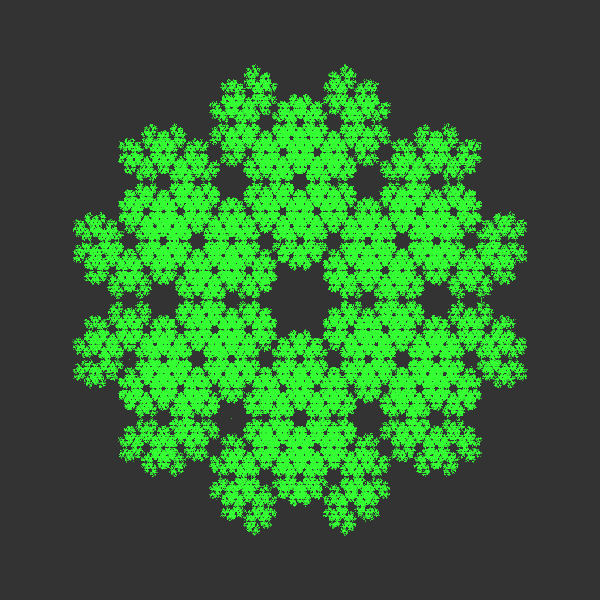If we use a different ALLOWED list [0, 1, 0, 1, 0, 1], then after using a particular vertex, the next vertex will have to be either the opposite or one of the two adjacent vertices. This is the result: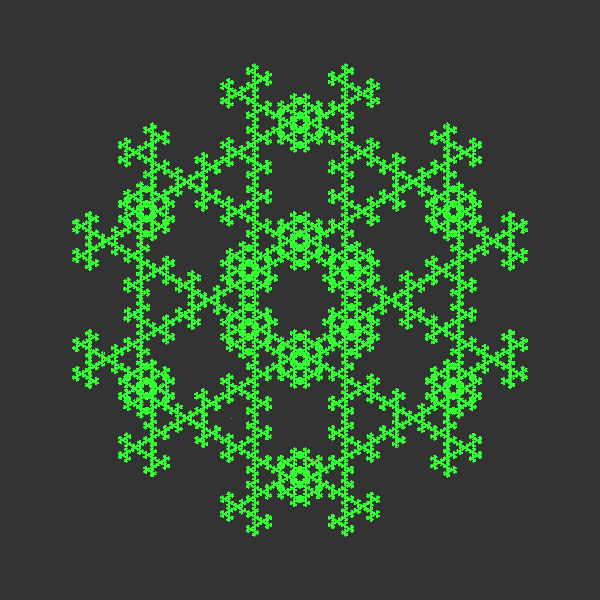You can try other shapes and allowed lists - you can also try irregular polygons.